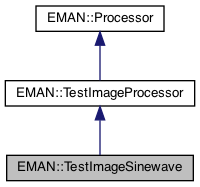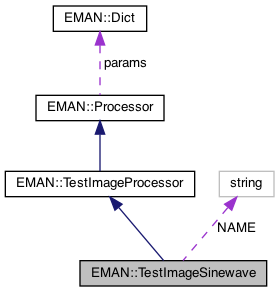EMAN2
EMAN::TestImageSinewave Class Reference

Replace a source image as a sine wave in specified wave length. More...

`#include <processor.h>`

Inheritance diagram for EMAN::TestImageSinewave:[legend]
Collaboration diagram for EMAN::TestImageSinewave:[legend]

## Public Member Functions

virtual void process_inplace (EMData *image)
To process an image in-place. More...

virtual string get_name () const
Get the processor's name. More...

virtual string get_desc () const
Get the descrition of this specific processor. More...

virtual TypeDict get_param_types () const
Get processor parameter information in a dictionary. More...Public Member Functions inherited from EMAN::Processor
virtual ~Processor ()

virtual EMDataprocess (const EMData *const image)
To proccess an image out-of-place. More...

virtual void process_list_inplace (vector< EMData * > &images)
To process multiple images using the same algorithm. More...

virtual Dict get_params () const
Get the processor parameters in a key/value dictionary. More...

virtual void set_params (const Dict &new_params)
Set the processor parameters using a key/value dictionary. More...

## Static Public Member Functions

static ProcessorNEW ()Static Public Member Functions inherited from EMAN::TestImageProcessor
static string get_group_desc ()Static Public Member Functions inherited from EMAN::Processor
static string get_group_desc ()
Get the description of this group of processors. More...

static void EMFourierFilterInPlace (EMData *fimage, Dict params)
Compute a Fourier-filter processed image in place. More...

static EMDataEMFourierFilter (EMData *fimage, Dict params)
Compute a Fourier-processor processed image without altering the original image. More...

## Static Public Attributes

static const string NAME = "testimage.sinewave"Public Types inherited from EMAN::Processor
enum  fourier_filter_types {
TOP_HAT_LOW_PASS , TOP_HAT_HIGH_PASS , TOP_HAT_BAND_PASS , TOP_HOMOMORPHIC ,
GAUSS_LOW_PASS , GAUSS_HIGH_PASS , GAUSS_BAND_PASS , GAUSS_INVERSE ,
GAUSS_HOMOMORPHIC , BUTTERWORTH_LOW_PASS , BUTTERWORTH_HIGH_PASS , BUTTERWORTH_HOMOMORPHIC ,
KAISER_I0 , KAISER_SINH , KAISER_I0_INVERSE , KAISER_SINH_INVERSE ,
SHIFT , TANH_LOW_PASS , TANH_HIGH_PASS , TANH_HOMOMORPHIC ,
}
Fourier filter Processor type enum. More...Protected Member Functions inherited from EMAN::TestImageProcessor
void preprocess (EMData *image)Protected Attributes inherited from EMAN::TestImageProcessor
int nx

int ny

int nzProtected Attributes inherited from EMAN::Processor
Dict params

## Detailed Description

Replace a source image as a sine wave in specified wave length.

Parameters
 wavelength wavelength in equation sin(x*2*PI/wavelength - phase*180/PI) axis (optional) specify a major axis for asymmetric features, default x axis phase (optional) the phase in radians az (optional) angle in degree. for 2D image, this is the rotated angle of the image, in 3D image, it's az for euler angle. default is zero alt (optional) angle in degree. only in 3D case, alt for euler angle, default is zero phi (optional) angle in degree. only in 3D case, phi for euler angle, default is zero

Definition at line 8523 of file processor.h.

## ◆ get_desc()

 virtual string EMAN::TestImageSinewave::get_desc ( ) const
inlinevirtual

Get the descrition of this specific processor.

This function must be overwritten by a subclass.

Returns
The description of this processor.

Implements EMAN::Processor.

Definition at line 8533 of file processor.h.

8534 {
8535 return "Replace a source image as a sine wave in specified wave length";
8536 }

## ◆ get_name()

 virtual string EMAN::TestImageSinewave::get_name ( ) const
inlinevirtual

Get the processor's name.

Each processor is identified by a unique name.

Returns
The processor's name.

Implements EMAN::Processor.

Definition at line 8528 of file processor.h.

8529 {
8530 return NAME;
8531 }
static const string NAME
Definition: processor.h:8556

References NAME.

Referenced by process_inplace().

## ◆ get_param_types()

 virtual TypeDict EMAN::TestImageSinewave::get_param_types ( ) const
inlinevirtual

Get processor parameter information in a dictionary.

Each parameter has one record in the dictionary. Each record contains its name, data-type, and description.

Returns
A dictionary containing the parameter info.

Reimplemented from EMAN::Processor.

Definition at line 8543 of file processor.h.

8544 {
8545 TypeDict d;
8546 d.put("wavelength", EMObject::FLOAT, "wavelength in equation sin(x*2*PI/wavelength - phase*180/PI)");
8547 d.put("axis", EMObject::STRING, "(optional) specify a major axis for asymmetric features, default x axis");
8548 d.put("phase", EMObject::FLOAT, "(optional) the phase in radians");
8549 d.put("az", EMObject::FLOAT, "(optional) angle in degree. for 2D image, this is the rotated angle of the image, \
8550 in 3D image, it's az for euler angle. default is zero");
8551 d.put("alt", EMObject::FLOAT, "(optional) angle in degree. only in 3D case, alt for euler angle, default is zero");
8552 d.put("phi", EMObject::FLOAT, "(optional) angle in degree. only in 3D case, phi for euler angle, default is zero");
8553 return d;
8554 }
TypeDict is a dictionary to store <string, EMObject::ObjectType> pair.
Definition: emobject.h:305
void put(const string &key, EMObject::ObjectType o, const string &desc="")
Definition: emobject.h:330

References EMAN::EMObject::FLOAT, EMAN::TypeDict::put(), and EMAN::EMObject::STRING.

## ◆ NEW()

 static Processor * EMAN::TestImageSinewave::NEW ( )
inlinestatic

Definition at line 8538 of file processor.h.

8539 {
8540 return new TestImageSinewave();
8541 }
Replace a source image as a sine wave in specified wave length.
Definition: processor.h:8524

## ◆ process_inplace()

 void TestImageSinewave::process_inplace ( EMData * image )
virtual

To process an image in-place.

For those processors which can only be processed out-of-place, override this function to just print out some error message to remind user call the out-of-place version.

Parameters
 image The image to be processed.

Implements EMAN::Processor.

Definition at line 9601 of file processor.cpp.

9602{
9603 preprocess(image);
9604
9605 if(!params.has_key("wavelength")) {
9606 LOGERR("%s wavelength is required parameter", get_name().c_str());
9607 throw InvalidParameterException("wavelength parameter is required.");
9608 }
9609 float wavelength = params["wavelength"];
9610
9611 string axis = "";
9612 if(params.has_key("axis")) {
9613 axis = (const char*)params["axis"];
9614 }
9615
9616 float phase = 0;
9617 if(params.has_key("phase")) {
9618 phase = params["phase"];
9619 }
9620
9621 int ndim = image->get_ndim();
9622 float * dat = image->get_data();
9623
9624 if(ndim==1) { //1D
9625 for(int i=0; i<nx; ++i, ++dat) {
9626 *dat = sin(i*(2.0f*M_PI/wavelength) + phase);
9627 }
9628 }
9629 else if(ndim==2) { //2D
9630 float alpha = 0;
9631 if(params.has_key("az")) {
9632 alpha = params["az"];
9633 }
9634 for(int j=0; j<ny; ++j) {
9635 for(int i=0; i<nx; ++i, ++dat) {
9636 if(alpha != 0) {
9637 *dat = sin((i*sin((180-alpha)*M_PI/180)+j*cos((180-alpha)*M_PI/180))*(2.0f*M_PI/wavelength) + phase);
9638 }
9639 else if(axis.compare("y")==0 || axis.compare("Y")==0) {
9640 *dat = sin(j*(2.0f*M_PI/wavelength) + phase);
9641 }
9642 else {
9643 *dat = sin(i*(2.0f*M_PI/wavelength) + phase);
9644 }
9645 }
9646 }
9647 }
9648 else { //3D
9649 float az = 0;
9650 if(params.has_key("az")) {
9651 az = params["az"];
9652 }
9653 float alt = 0;
9654 if(params.has_key("alt")) {
9655 alt = params["alt"];
9656 }
9657 float phi = 0;
9658 if(params.has_key("phi")) {
9659 phi = params["phi"];
9660 }
9661
9662 for(int k=0; k<nz; ++k) {
9663 for(int j=0; j<ny; ++j) {
9664 for(int i=0; i<nx; ++i, ++dat) {
9665 if(axis.compare("z")==0 || axis.compare("Z")==0) {
9666 *dat = sin(k*(2.0f*M_PI/wavelength) + phase);
9667 }
9668 else if(axis.compare("y")==0 || axis.compare("Y")==0) {
9669 *dat = sin(j*(2.0f*M_PI/wavelength) + phase);
9670 }
9671 else {
9672 *dat = sin(i*(2.0f*M_PI/wavelength) + phase);
9673 }
9674 }
9675 }
9676 }
9677
9678 if(az != 0 || alt != 0 || phi != 0) {
9679 Dict d("type","eman");
9680 d["az"] = az; d["phi"] = phi; d["alt"] = alt;
9681 image->transform(Transform(d));
9682 }
9683 }
9684
9685 image->update();
9686}
Dict is a dictionary to store <string, EMObject> pair.
Definition: emobject.h:385
bool has_key(const string &key) const
Ask the Dictionary if it as a particular key.
Definition: emobject.h:511
void transform(const Transform &t)
Rotate then translate the image.
Definition: emdata.h:295
void preprocess(EMData *image)
Definition: processor.cpp:8923
virtual string get_name() const
Get the processor's name.
Definition: processor.h:8528
A Transform object is a somewhat specialized object designed specifically for EMAN2/Sparx storage of ...
Definition: transform.h:75
EMData * phase() const
return phase part of a complex image as a real image format
#define InvalidParameterException(desc)
Definition: exception.h:361
#define LOGERR
Definition: log.h:51

## ◆ NAME

 const string TestImageSinewave::NAME = "testimage.sinewave"
static

Definition at line 8556 of file processor.h.

Referenced by get_name().

The documentation for this class was generated from the following files: